# Web开发应该知道的数据结构

## 数据结构概念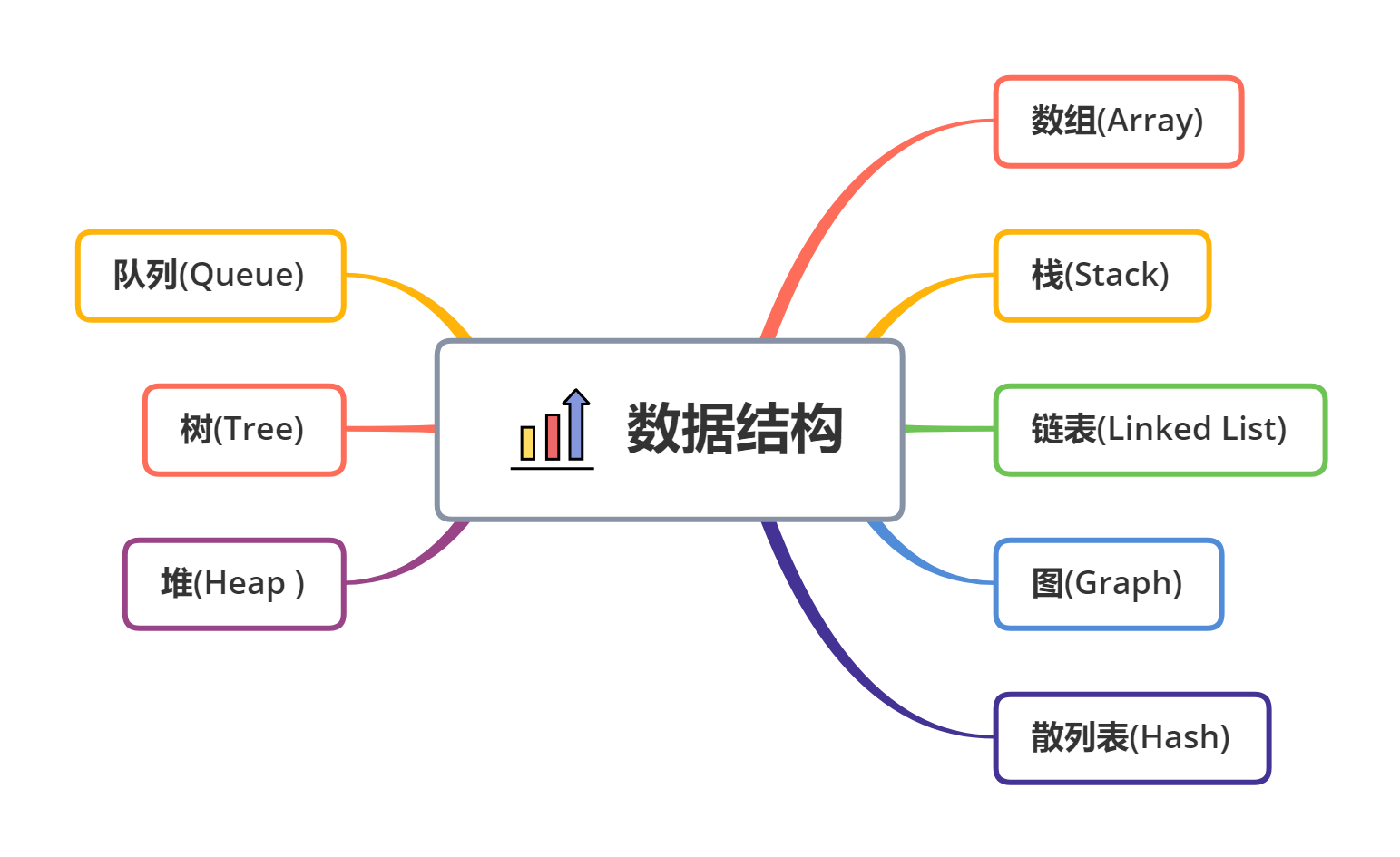## 数组 🌈

### 定义

• 优点
1. 按照索引查询元素速度快
2. 按照索引遍历数组方便
• 缺点
1. 数组的大小固定后就无法扩容了
2. 数组只能存储一种类型的数据
• 扩展
1. JavaScript数组中的默认存储值是undefined，其它编程语言数组的默认存储值是0或者是垃圾数据
2. 与其它的编程语言不同，JavaScript可以访问数组中不存在的索引，会返回undefined，而其它的编程语言会报错或返回垃圾数据
3. JavaScript可以存储不同类型的数据，而其它的编程语言只能存储一种数据类型的数据
4. 当JavaScript中数组的存储空间不够用时，它会自动扩容，而其它的语言数组的大小是固定的，一旦定义了，就无法改变
5. JavaScript中分配给数组的存储空间是不连续的，而其他编程语言中分配给数组的存储空间是连续的
• 适用场景

## 栈 🎯

### 定义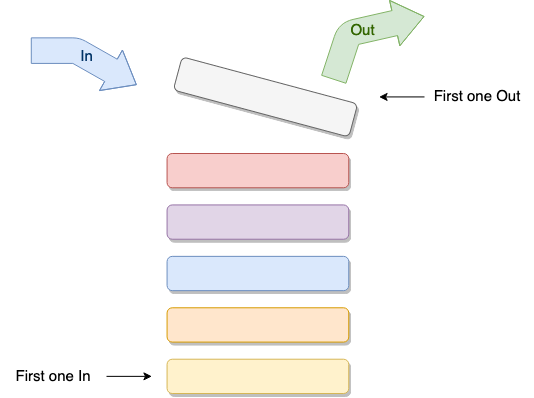• 适用场景

### 实现

``````module.exports =  class Stack {
data = []
maxSize

constructor(initialData, maxSize = -1) {
this.data = Array.isArray(initialData) ? initialData : (typeof initialData == "undefined" ? [] : [initialData])
this.maxSize = maxSize
}

isFull() {
return this.maxSize != -1 ? (this.data.length == this.maxSize) : false
}

isEmpty() {
return this.data.length == 0
}

add(item) {
if(this.isFull()) {
return false
}
this.data.push(item)
}

*generator() {
while(!this.isEmpty()) {
yield this.data.pop()
}
}

pop() {
const { value, done } = this.generator().next()
if(done) return false
return value
}
}

``````const Stack = require("./stack.js")
class Operation {
constructor(val) {
this.value = val
}
}
class Add extends Operation {
apply(value) {
return value + this.value
｝
undo(value) {
return value - this.value
}
}
class Times extends Operation {
apply(value) {
return value * this.value
}
undo(value) {
return value / this.value
}
}
/** 操作栈 **/
class OpsStack {
constructor() {
this.value = 0
this.operations = new Stack()
}
add(op) {
this.value = op.apply(this.value)
this.operations.add(op)
}

undo() {
if(this.operations.isEmpty()) {
return false
}
this.value = (this.operations.pop()).undo(this.value)
}
}
let s = new OpsStack()
s.add(new Add(1))
s.add(new Add(1))
s.add(new Times(2))
console.log("Current value: ", s.value)
s.undo()
s.undo()
console.log("Final value: ", s.value
// Current value:  4
// Final value:  1

## 队列 ✍

### 定义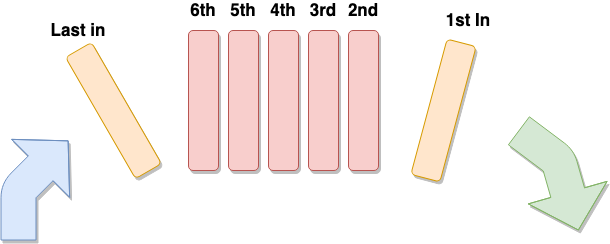• 适用场景因为队列先进先出的特点，在多线程阻塞队列管理中非常适用。

### 实现

``````class Queue {
data = [];
maxSize;

constructor(initialData, maxSize = -1) {
this.data = Array.isArray(initialData) ? initialData : typeof initialData == 'undefined' ? [] : [initialData];
this.maxSize = maxSize;
}

isFull() {
return this.maxSize != -1 ? this.data.length == this.maxSize : false;
}

isEmpty() {
return this.data.length == 0;
}

enqueue(item) {
if (this.isFull()) {
return false;
}
this.data.push(item);
}

*generator() {
while (!this.isEmpty()) {
yield this.data.shift();
}
}

dequeue() {
const { value, done } = this.generator().next();
if (done) return false;
return value;
}
}

``````const  Queue  = require('./queues')

let q = new Queue(3, 2)

q.enqueue(1)
q.enqueue(2)

let x = 0
while(x = q.dequeue()) {
console.log(x)
}
/*
Prints:
3
1
*/

• 优先级队列：其元素在内部按优先级值排序。
• 循环队列：其最后一个元素指向第一个。
• 双端队列：一种具有队列和栈的性质的数据结构。双端队列中的元素可以从两端弹出（这听起来像作弊！）。

## 堆 🚀

### 定义

• 特点
1. 任一结点的值是其子树所有结点的最大值或最小值
2. 必须是完全二叉树
• 适用场景

### 实现

1. 在这里先将元素 5 插入到末尾，即放在结点 6 的右子树。
2. 然后与父类比较， 6 > 5 ，父类数字大于子类数字，子类与父类交换。
3. 重复此操作，直到不发生替换。

`核心点：将最后一个元素填充到堆顶，然后不断的下沉这个元素。`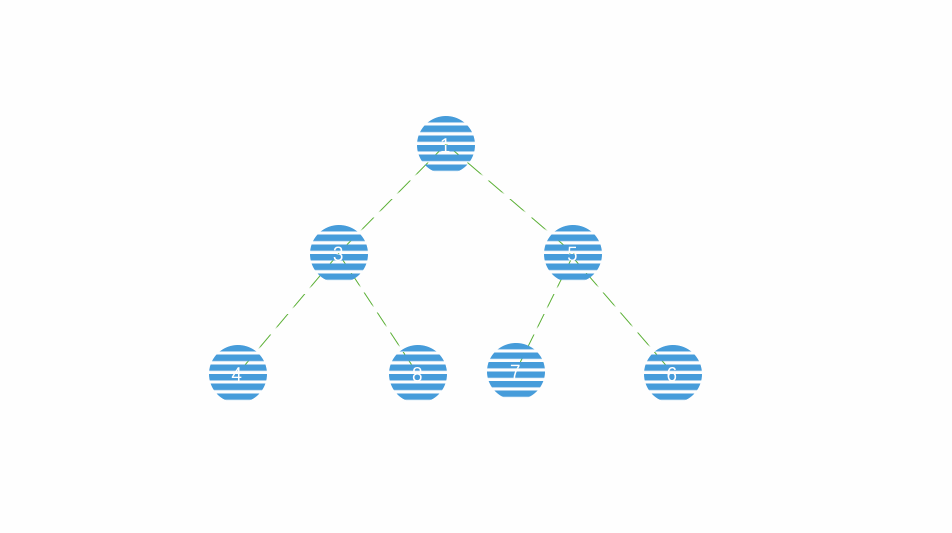• 找到要删除的节点（取出的节点）在数组中的位置
• 用数组中最后一个元素替代这个位置的元素
• 当前位置和其左右子树比较，保证符合最小堆的节点间规则
• 删除最后一个元素

## 链表 🏉

### 定义

• 优点
1. 链表是很常用的一种数据结构，不需要初始化容量，可以任意加减元素；
2. 添加或者删除元素时只需要改变前后两个元素结点的指针域指向地址即可，所以添加，删除很快；
• 缺点
1. 因为含有大量的指针域，占用空间较大；
2. 查找元素需要遍历链表来查找，非常耗时。
• 适用场景

• 循环链表的特点

• 双向链表的特点
1. 与单链表对比，双链表需要多一个指针用于指向前驱节点，因此如果存储同样多的数据，双向链表要比单链表占用更多的内存空间
2. 双链表的插入和删除需要同时维护 next 和 prev 两个指针。
3. 双链表中的元素访问需要通过顺序访问，支持双向遍历，这就是双向链表操作的灵活性根本

### 实现

1. 添加元素

`尾插法：`将链表的左边称为链表头部，右边称为链表尾部。尾插法是将左边固定，每次新增都在链表的右边最尾部。

1. 查询元素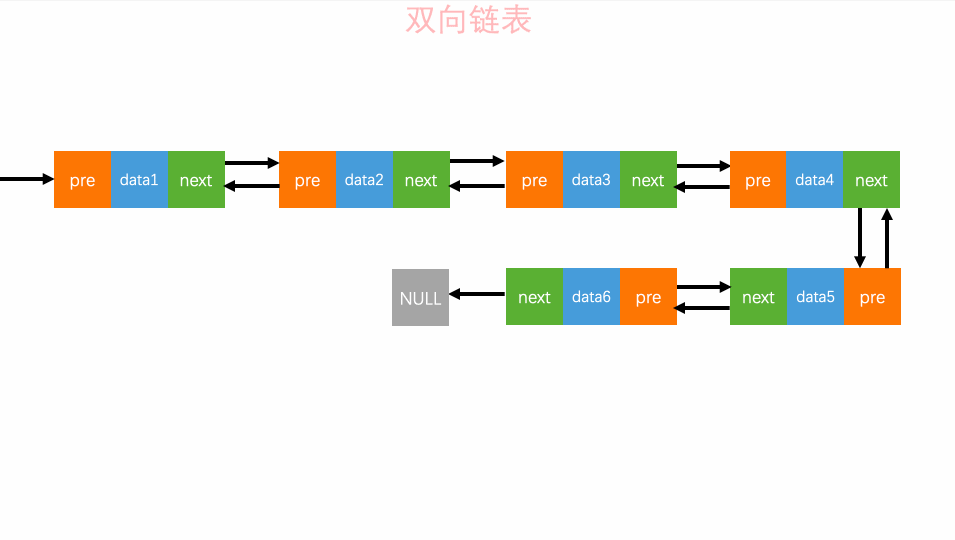双向链表的灵活处就是`知道链表中的一个元素结构就可以向左或者向右开始遍历查找需要的元素结构。`因此对于一个有序链表，双向链表的按值查询的效率比单链表高一些。因为，我们可以记录上次查找的位置 p，每次查询时，根据要查找的值与 p 的大小关系，决定是往前还是往后查找，所以平均只需要查找一半的数据。

1. 删除元素

• 删除结点中“值等于某个给定值”的结点
• 删除给定指针指向的结点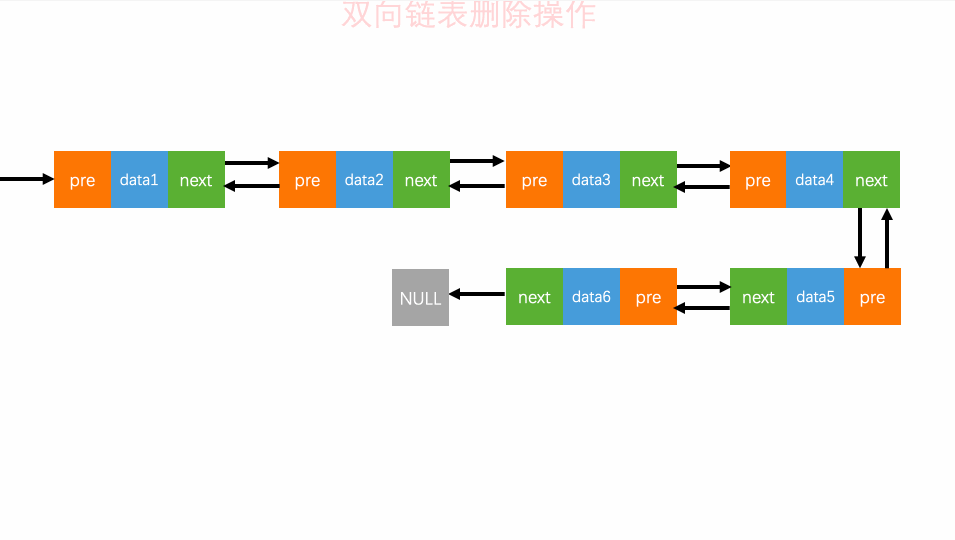对于双向链表来说，双向链表中的结点已经保存了前驱结点的指针，删除时不需要像单链表那样遍历。所以，针对第二种情况，单链表删除操作需要 O(n) 的时间复杂度，而双向链表只需要在 O(1) 的时间复杂度。 4. 双向循环链表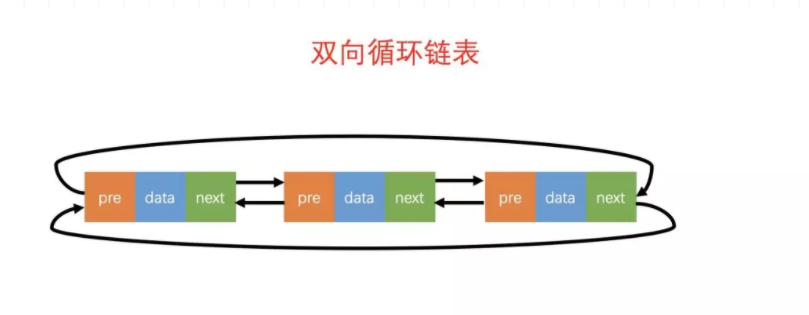如图所示，双向循环链表的概念很好理解：「双向链表」 + 「循环链表」的组合。

## 树 🏈

### 定义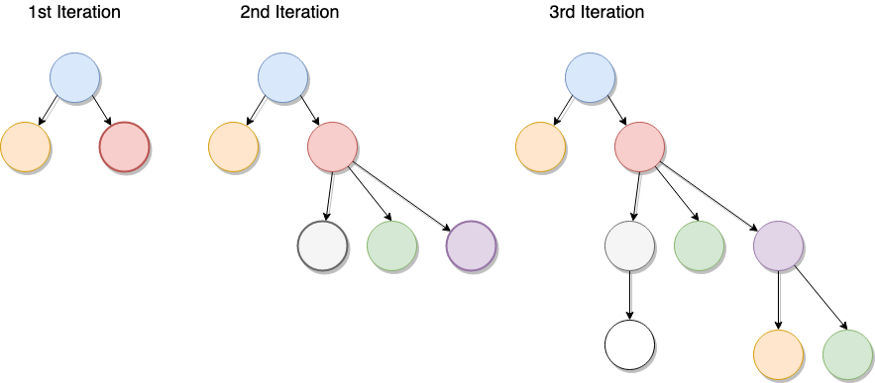• 特点
1. 每个节点有零个或多个子节点；
2. 没有父节点的节点称为根节点；
3. 每一个非根节点有且只有一个父节点；
4. 除了根节点外，每个子节点可以分为多个不相交的子树；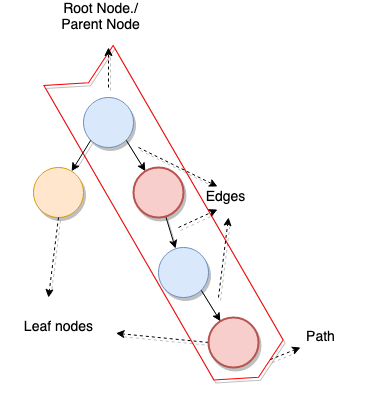树的不同部分被称为：

• 根节点/父节点(Root Node/parent Node)：在其下有子节点的节点。
• 叶节点(leaf nodes)：没有与其关联的子节点的节点。
• 边(edges)：两个节点之间的链接。

• 路径：从根节点到目标节点所需的节点列表。
• 树的高度：在根节点和最远的叶节点之间形成最大路径的节点数。

• 二叉树：二叉树是树的特殊一种，它添加，删除元素都很快，并且在查找方面也有很多的算法优化，所以，二叉树既有链表的好处，也有数组的好处，是两者的优化方案，在处理大批量的动态数据方面非常有用，它们还用于创建二进制搜索树。他的的一种潜在用例是压缩算法。具有如下特点：
1. 每个结点最多有两颗子树，结点的度最大为2。
2. 左子树和右子树是有顺序的，次序不能颠倒。
3. 即使某结点只有一个子树，也要区分左右子树。
• 二分搜索树：也称为二叉搜索树、有序二叉树（ordered binary tree）或排序二叉树（sorted binary tree），是指一棵空树或者具有下列性质的二叉树
1. 若任意节点的左子树不空，则左子树上所有节点的值均小于它的根节点的值；
2. 若任意节点的右子树不空，则右子树上所有节点的值均大于或等于它的根节点的值；
3. 任意节点的左、右子树也分别为二叉查找树；
• 深度优先搜索（DFS）：这是查找树的一种方式。它的工作方式是，首先先遍历整个左侧，然后回溯到最后访问的父级，然后移至右侧的子树。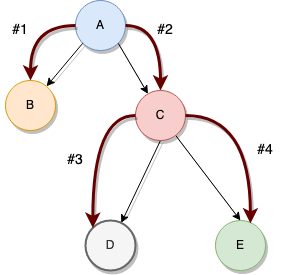### 实现

``````class BinaryTreeNode {
constructor(value) {
this.value = value;
this.left_child = null;
this.right_child = null;
}
compare(v) {
if (this.value > v) return -1;
if (this.value == v) return 0;
if (this.value < v) return 1;
}
}
class BST {
constructor() {
this.root_node = null;
}
/* 如果根节点为空（树为空），则elem将成为根节点

add(elem) {
if (!this.root_node) {
this.root_node = new BinaryTreeNode(elem);
return;
}
let inserted = false;
let currentNode = this.root_node;
do {
let comp = currentNode.compare(elem);
if (comp == -1) {
if (!currentNode.left_child) {
currentNode.left_child = new BinaryTreeNode(elem);
inserted = true;
} else {
currentNode = currentNode.left_child;
}
}
if (comp != -1) {
if (!currentNode.right_child) {
currentNode.right_child = new BinaryTreeNode(elem);
inserted = true;
} else {
currentNode = currentNode.right_child;
}
}
} while (!inserted);
}
inorder(parent) {
if (parent) {
this.inorder(parent.left_child);
console.log(parent.value);
this.inorder(parent.right_child);
}
}
print() {
this.inorder(this.root_node);
}
}

``````const Tree = require("./bst")
const t = new Tree()
t.add(10)
t.add(8)
t.add(11)
t.add(23)
t.add(1)
t.add(9)
t.print()
/*
Prints:
1
8
9
10
11
23
*/

## 图 🏂

### 定义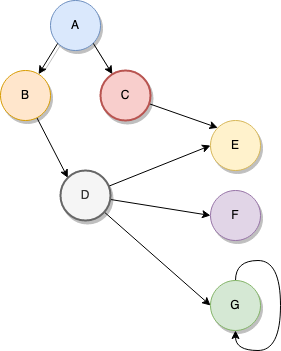按照顶点指向的方向可分为无向图和有向图： 图是一种比较复杂的数据结构，在存储数据上有着比较复杂和高效的算法，分别有邻接矩阵 、邻接表、十字链表、邻接多重表、边集数组等存储结构

• 适用场景

### 实现

``````class Node {

constructor(value) {
this.value = value
this.links = []
}

linkTo(node, weight) {
this.links.push(new Link(this, weight, node))
}
}

class Link {

constructor(a, weight, b) {
this.left = a;
this.weight = weight
this.right = b
}
}

class Graph {

constructor(root_node) {
this.root_node = root_node
this.dfs_visited = new Set();
}

dfs(starting_node) {
if(!starting_node) starting_node = this.root_node
let node = starting_node
console.log(node.value);
this.dfs_visited.add(node);
node.links.forEach( neighbour => {
if (!this.dfs_visited.has(neighbour.right)) {
this.dfs(neighbour.right);
}
})
}

}

``````//定义节点
let A = new Node("A")
let B = new Node("B")
let C = new Node("C")
let D = new Node("D")
let E = new Node("E")
let F = new Node("F")
let G = new Node("G")

//定义节点间的关系
A.linkTo(B, 1)
A.linkTo(C, 2)
B.linkTo(D, 1)
C.linkTo(E, 10)
D.linkTo(E, 10)
D.linkTo(F, 1)
D.linkTo(G, 1)
G.linkTo(G, 1)

let g = new Graph(A)
//遍历图表
g.dfs()

## 散列表 🎉

### 定义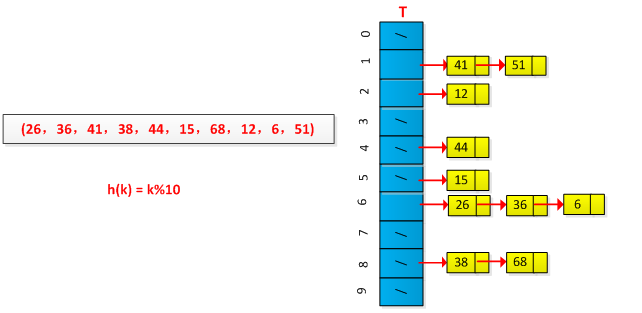记录的存储位置=f(key)

• 优点
1. 利用hash表的优势，对于集合的查找元素时非常方便；
• 缺点
1. 因为哈希表是基于数组衍生的数据结构，在添加删除元素方面是比较慢的；
• 适用场景

### 实现

``````class HashMap {

constructor() {
this.map = {}
}

hash(k) {
return k % 10
}

add(key, value) {
let k = this.hash(key)
if(!this.map[k]) {
this.map[k] = []
}
this.map[k].push(value)
}

get(key) {
let k = this.hash(key)
return this.map[k]
}

}

let h = new HashMap()

h.add(10, "hello")
h.add(100001, "world")
h.add(1, "this is a string")

console.log(h)

`输出结果如下`

``````HashMap {
map: { '0': [ 'hello' ], '1': [ 'world', 'this is a string' ] }
}

## 参考文章 📜

LeetCodeAnimation

Data Structures You Should Know as a JavaScript Developer